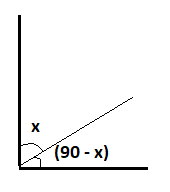QUESTION

# If two complementary angles differ by ${12^0}$, then what will be the value of angles ?

Hint: Two angles are complementary if their sum is equal to ${90^0}$. So, let us assume any angle x then its complement will be 90 – x. Now we form a linear equation by using a given condition(difference of two angles is ${12^0}$). And then solve this equation to get the required value of x.

If two angles are complementary, then sum of their measures is ${90^0}$

So, let one of the complementary angles be ${x^0}$

This means that its complement will be ${90^0}$–${x^0}$.

But as given in the question that the difference in their measures is ${12^0}$.

Therefore, you can write the equation:

${x^0} - \left( {{{90}^0} - {x^0}} \right) = {12^0}$ (1)

Since the parentheses are preceded by a minus sign, so if we remove the parentheses then we have to also change the signs of the terms inside the parentheses. Therefore, when we remove the parentheses then equation becomes:

${x^0} - {90^0} + {x^0} = {12^0}$

Combine the two terms $\left( {{x^0}} \right)$ on the left side of the above equation. We get,

$2{x^0} - {90^0} = {12^0}$

To get rid of the ${90^0}$ on the left side. We add ${90^0}$ to the both sides of the equation.

$2{x^0} = {102^0}$

Now to find the value of x we divide both sides of the above equation by 2.

${x^0} = {51^0}$

So, the measure of one of the two angles is ${51^0}$. So, its complement is the amount that
must be added to that angle such that this sum is ${90^0}$. By subtracting ${51^0}$ from ${90^0}$, we will find that the complement of the angle is ${39^0}$.

Hence, the measures of the two complementary angles whose difference is equal to ${12^0}$ are ${51^0}$and ${39^0}$.Note: Whenever we come up with this type of problem then we should remember that the sum of two complementary angles is 90 degrees. And the sum of two supplementary angles is equal to ${180^0}$. So, if we are given any condition for complementary angles and asked to find the angles. So, we assume any one angle as x and another angle as 90 – x. And then apply the given condition to get the value of x.